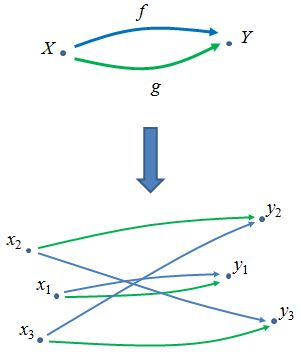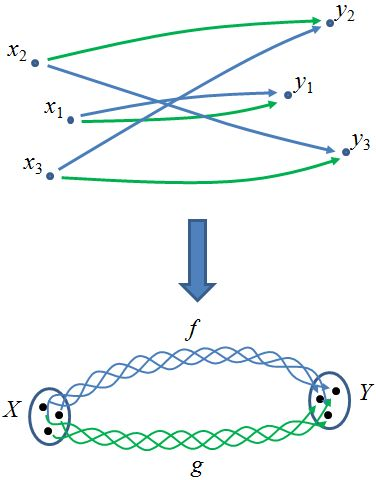Contents

category theory

# Contents

## Idea

The category of elements of a functor $F : C \to$ Set is a category $el(F) \to C$ sitting over the domain category $C$, such that the fiber over an object $c \in C$ is the set $F(c)$.

This is a special case of the Grothendieck construction, by considering sets as discrete categories.

We may think of Set as the classifying space of “Set-bundles;” see generalized universal bundle. The category of elements of $F$ is, in this sense, the Set-bundle classified by $F$. It comes equipped with a projection to $C$ which is a discrete opfibration, and provides an equivalence between $Set$-valued functors and discrete opfibrations. (There is a dual category of elements that applies to contravariant $Set$-valued functors, i.e. presheaves, and produces discrete fibrations.)

Forming a category of elements can be thought of as “unpacking” a concrete category. For example, consider a concrete category $C$ consisting of two objects $X,Y$ and two non-trivial morphisms $f,g$The individual elements of $X,Y$ are “unpacked” and become objects of the new category. The “unpacked” morphisms are inherited in the obvious way from morphisms of $C$.

Note that an “unpacked” category of elements can be “repackaged”.The generalization of the category of elements for functors landing in Cat, rather than just $Set$, is called the Grothendieck construction.

## Definition

Given a functor $P:C\to\mathbf{Set}$, the category of elements $el(P)$ or $El_P(C)$ (or obvious variations) may be understood in any of these equivalent ways:

• It is the category whose objects are pairs $(c,x)$ where $c$ is an object in $C$ and $x$ is an element in $P(c)$ and morphisms $(c,x)\to(c',x')$ are morphisms $u:c\to c'$ such that $P(u)(x) = x'$.

• It is the pullback along $P$ of the universal Set-bundle $U : Set_* \to Set$

$\array{ El_P(C) &\to& Set_* \\ \downarrow^{\mathrlap{\pi_P}} && \downarrow^\mathrlap{U} \\ C &\to& Set }\,,$

where $U$ is the forgetful functor from pointed sets to sets.

• It is the comma category $(*/P)$, where $*$ is the inclusion of the one-point set $*:*\to Set$ and $P:C\to Set$ is itself:

$\array{ El_P(C) &\to& * \\ \downarrow^{\mathrlap{\pi_P}} &\Downarrow& \downarrow^{\mathrlap{pt}} \\ C &\to& Set }$
• Its opposite is the comma category $(Y/P)$, where $Y$ is the Yoneda embedding $C^{op}\to [C,Set]$ and $P$ is the functor $*\to [C,Set]$ which picks out $P$ itself:

$\array{ El_P(C)^{op} &\overset{\pi_P^{op}}{\to}& C^{op} \\ \downarrow &\Downarrow& \downarrow^{\mathrlap{Y}} \\ * & \underset{P}{\to}& [C,Set] }$

$El_P(C)$ is also often written with coend notation as $\int^C P$, $\int^{c: C} P(c)$, or $\int^c P(c)$. This suggests the fact the set of objects of the category of elements is the disjoint union (sum) of all of the sets $P(c)$.

• It is the (strict) oplax colimit of the composite $C \xrightarrow{P} \mathbf{Set} \xrightarrow{disc} \mathbf{Cat}$; see Grothendieck construction.

When $C$ is a concrete category and the functor $F:C\to Set$ is simply the forgetful functor, we can define a functor

$Explode(-) := El_F(-).$

This is intended to illustrate the concept that constructing a category of elements is like “unpacking” or “exploding” a category into its elements.

## Properties

The category of elements defines a functor $el : \mathbf{Set}^{C} \to \mathbf{Cat}$. This is perhaps most obvious when viewing it as an oplax colimit. Furthermore we have:

###### Theorem

The functor $el : \mathbf{Set}^{C} \to \mathbf{Cat}$ is cocontinuous.

###### Proof

As remarked above, $el$ is a strict weighted 2-colimit, hence we have an isomorphism

$el(P) \cong \int^{c\in C} J(c) \times disc(P(c))$

where the weight $J:C^{op} \to \mathbf{Cat}$ is the functor $c\mapsto c/C$, and $disc:\mathbf{Set}\hookrightarrow \mathbf{Cat}$ is the inclusion of the discrete categories. But since $disc$ (regarded purely as a 1-functor) has a right adjoint (the functor which sends a -small- category $C$ into its set of elements $C_0$), it preserves (1-categorical) colimits. Since colimits also commute with colimits, the composite operation $\el$ also preserves colimits.

###### Theorem

The functor $el\colon \mathbf{Set}^{C} \to \mathbf{Cat}$ has a right adjoint (which is maybe a more direct way to see that it is cocontinuous).

###### Proof

By a simple coend computation

$\begin{array}{rl} \mathbf{Cat}(el(F),D)&\cong \mathbf{Cat}\Big( \int^c J c\times\delta(F c), D\Big)\\ &\cong \int_c\mathbf{Cat}\big(J c\times \delta(F c),D\big)\\ &\cong \int_c \mathbf Sets\big(F c,[J c,D]_0\big)\\ &\cong \mathbf{Set}^{C}(F, K(D)) \end{array}$

where $K(D)\colon c\mapsto [J c,D]_0$.

Now for any $C$, the terminal object of $\mathbf{Set}^C$ is the functor $\Delta 1$ constant at the point. The category of elements of $\Delta 1$ is easily seen to be just $C$ itself, so the unique transformation $P\to \Delta 1$ induces a projection functor $\pi_P: \el(P) \to C$ defined by $(c,x)\mapsto c$ and $u\mapsto u$. The projection functor is a discrete opfibration, and can be viewed also as a $C$-indexed family of sets. When we regard $\el(P)$ as equipped with $\pi_P$, we have an embedding of $\mathbf{Set}^C$ into $\mathbf{Cat}/C$.

Note that while the canonical projection $\operatorname{El}(F) \to \mathbf{C}$ is surjective on objects, it is not usually full. For example, let $\mathbf{B}\mathbb{N}$ be the one-object category which carries the monoid $(\mathbb{N}, +)$ as its endomorphism monoid, and let $F$ be the action of $(\mathbb{N}, +)$ on the set $\mathbb{N}$ by $n.m = m + n$. Then the image of any hom-set between $k, k'$ is a singleton subset of $\mathbb{N}$.

More generally, the universal covering groupoid of a groupoid is just the category of elements of its action on itself by composition. Since this action is faithful and transitive, hom-sets in the category of elements are always $0$ or $1$, while objects in the groupoid might have nontrivial automorphism groups.

## Examples

### Representable Presheaves

Let $Y(C):\mathcal{C}^{op}\to Set$ be a representable presheaf with $Y(C)(D)=Hom_{\mathcal{C}}(D,C)$. Consider the contravariant category of elements $\int_\mathcal{C} Y(C)$ . This has objects $(D_1,p_1)$ with $p_1\in Y(C)(D_1)$, hence $p_1$ is just an arrow $D_1\to C$ in $\mathcal{C}$. A map from $(D_1, p_1)$ to $(D_2, p_2)$ is just a map $u:D_1\to D_2$ such that $p_2\circ u =p_1$ but this is just a morphism from $p_1$ to $p_2$ in the slice category $\mathcal{C}/C$. Accordingly we see that $\int_\mathcal{C} Y(C)\simeq \mathcal{C}/C$ .

This equivalence comes in handy when one wants to compute slices of presheaf toposes over representable presheaves $Y(C)$ since $PSh(\int_\mathcal{C} P) \simeq PSh(\mathcal{C})/P$ in general for presheaves $P:\mathcal{C}^{op}\to Set$ , whence $PSh(\mathcal{C})/Y(C) \simeq PSh(\mathcal{C}/C)$ . An instructive example of this construction is spelled out in detail at hypergraph.

### Action Groupoid

In the case that $C = \mathbf{B}G$ is the delooping groupoid of a group $G$, a functor $\varrho : \mathbf{B}G \to Set$ is a permutation representation $X$ of $G$ and its category of elements is the corresponding action groupoid $X/\!/G$.

###### Proof

This is easily seen in terms of the characterization $el(\varrho)\cong (*/\varrho)$, the category having as objects triples $(*,*; *\to \varrho(*)=X)$, namely elements of the set $X=\varrho(*)$, and as arrows $x\to y$ those $g\in \mathbf{B}G$ such that

$\array{ {*} & \overset{x}{\to} & X \\ {}^1\downarrow && \downarrow^g\\ {*} & \underset{y}{\to} & X }$

commutes, namely $g . x=\varrho(g)(x)=y$. We can also present the right adjoint to $el(-)$: one must consider the functor $J\colon \mathbf{B}G^{op}\to \mathbf{Cat}$, which represents $G$ in $\mathbf{Cat}$, and sends the unique object $*\in \mathbf{B}G$ to $*/\mathbf{B}G\cong G/\!/G$, the left action groupoid of $G$. The functor $J$ sends $h\in G$ to an automorphism of $G/\!/G$, obtained multiplying on the right $x\to g x$ to $x h\to x g h$.

Now for any category $D$, $K( D)(*)$ is exactly the set of functors $[G/\!/G, D]$, which inherits from $G/\!/G$ an obvious action: given $F\in [G/\!/G, D]$ we define $F^h=J(h)^*F=F \circ J(h) \colon g \mapsto F(g h)$.

### Category of simplices

For a simplicial set regarded as a presheaf on the simplex category, the corresponding category of elements is called its category of simplices. See there for more.

## Reference

A very nice introduction emphasizing the connections to monoid theory is ch. 12 of

Last revised on September 15, 2021 at 11:03:22. See the history of this page for a list of all contributions to it.